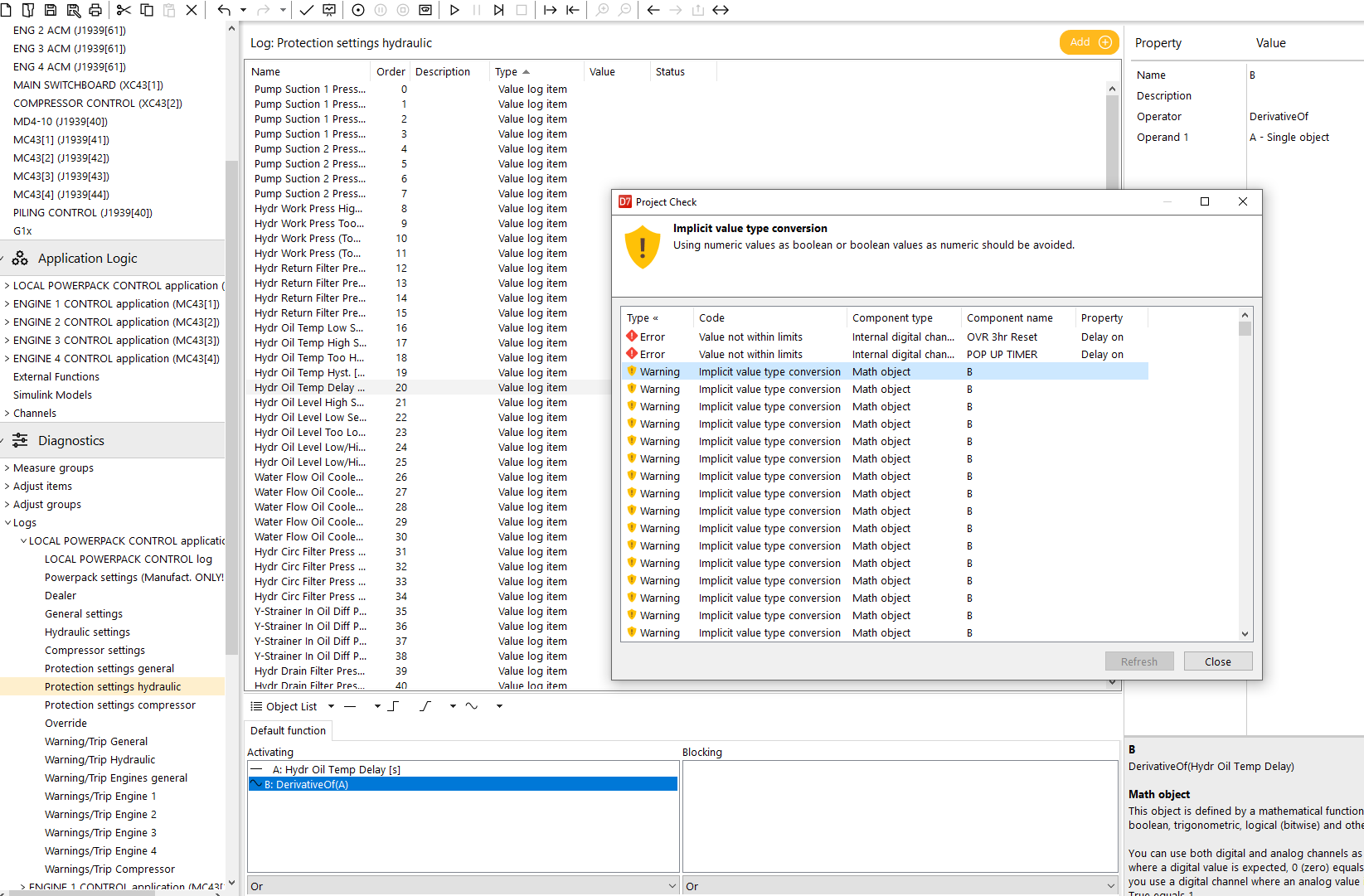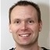0

# Impicit value type conversion

Grisel, Evert-Jan 4 weeks ago in IQANdesign updated by Gustav Widén (System support) 2 weeks ago

Since version 7 I get a "Impicit value type conversion" warning when I use a Derivative function in the activation part of a value log item.

Does anyone have a idea how to solve this?In the help is stated that it is possible to use the Derivative function as a digital:

You can use both digital and analog channels as operands. If you use an analog channel where a digital value is expected, 0 (zero) equals False and any other value equals True. If you use a digital channel where an analog value is expected, False equals 0 (zero) and True equals 1.Checking rising and falling edge on Boolean channels, you can use the functions Flank, PositiveFlank and NegativeFlank instead.I want to log the value if someone change this value, so it's not a boolean value which is changing.

Do you mean that I have to use a Flank function on a analog value?Sorry, I did not look close enough on the printscreen.

For detecting if a value increased, you can use DerivativeOf(value) > 0

And vice versa, for detecting if a value increased, you can use DerivativeOf(value) < 0

For detecting if a value changed in the last cycle, you can use DerivativeOf(value) <> 0

Compared to the implicit conversion you have now, this does add another calculation object to your expression.

But looking at the example printscreen, you can also save an unnecessary single object by having the DerivativeOf() function look directly at the value.